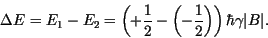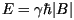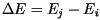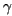Fabricant d'électro-aimants,
de teslamètres RMN,
d'alimentations de puissanceFabricant d'électro-aimants, de teslamètres RMN, d'alimentations de puissance# NMR Principle

According to the alignment of the spin there is an additional potential energy term:wheredenotes the magnetic field.

As the alignment of the nuclear spinis quantised there is a discrete set of additional energy levels Ei the nucleus can occupy for a certain magnetic field. Thus, the energy states of particles with spin 1/2, like the proton, which have opposed spin alignment differ by:The energy state of the nucleus can be altered by an external electromagnetic force, for example by a resonant circuit. The interaction between the nucleus and the source of the external force is mediated by the photon, the quantum of the electromagnetic interaction. As the energy level of the nucleus is quantised a nucleus with the initial energy Ei can only absorb or emit photons of the energy:A proton for example can only emit or absorb photons of the energy:According to Planck this corresponds to an electromagnetic wave of the frequency:where h is Planck's constant. This frequency is called Larmor frequency.

The frequency depends on the magnetic field and the gyromagnetic ratio.

Thus knowing the gyromagnetic ratio a measurement of the frequency whereat absorption occurs can be used to measure the magnetic field strength. As result of the thermal motion of the nuclei, this frequency is broadened due to the Doppler effect. So absorption occurs within a small band.

The measurement is performed with the help of a probe placed inside the magnetic field. The probe consists of resonant material which is surrounded by a resonant circuit. For magnetic fields up to 2T protons are used as resonant material. Deuterium is used for stronger fields.

The operation frequency of the resonant circuit, which corresponds to the frequency of the emitted photons, is varied. When emitted photons get absorbed by the resonant material the circuit releases energy. This loss is detected. The frequency of maximal absorption is searched. Here, the circuit operates at Larmor's frequency. The frequency can be measured to a high accuracy and can be used to calculate the magnetic field strength.

The error in the value of the magnetic field is that of the gyromagnetic ratio.

The error of measurement of the Larmor frequency is negligible.

The accuracy of the gyromagnetic ratio of proton and deuterium allows an accuracy better than 0.5 microTesla. Thus, NMR gaussmeters are the most accurate means of magnetic field measurement.

Author: Götz Gaycken, Hamburg, 1998.